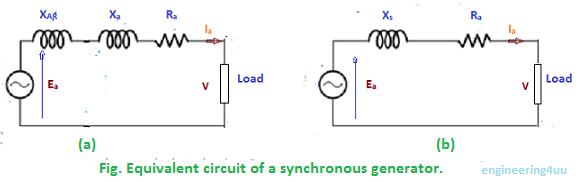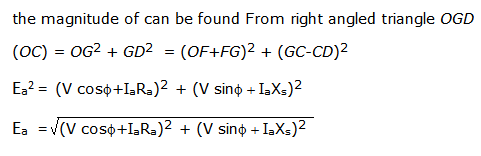The equivalent circuit of synchronous generator in show in fig.(a) .it is redrawn in fig (b) by taking Xs = XAR + XaLagging Power Factor cos Φ :
Fig shows the phasor diagram for lagging load. the power factor is cosΦ lagging. In this diagram the terminal voltage V is taken as reference phasor along OA such that OA = V. For lagging  power factor cos Φ the direction of the armature current Ia lags behind V by angle f along OB , where OB = Ia. the voltage drop in the armature resistance is Ia Ra .  It is represented by phasor ACThe voltage drop in synchronous reactance is Ia Xs . ti is represented by CD. it leads the current Ia by  90 and , therefore CD drawn in a direction perpendicular to OB the total voltage drop in the synchronous impedance is the phasor sum Ia Ra and Ia Xs . ti is represented by AD. the phasor OD represents Ea.Unity Power Factor :
The phasor diagram for unity power factor is shown in figure.Leading Power Factor cos Φ :
The phasor diagram for leading power factor is shown in figure.the angle between Ea and V is called the power angle or torque angle of the machine.It varies with load and is a measure of air gap power developed in the machine.